# Grothendieck group

(diff) ← Older revision | Latest revision (diff) | Newer revision → (diff)

An Abelian group that is assigned to an additive category by a universal additive mapping property. More exactly, letbe a small additive category and letbe an Abelian group. A mappingis said to be additive if for any exact sequence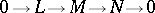of objects fromthe relation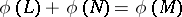is valid. There exists a group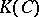, called the Grothendieck group of, and an additive mapping, known as the universal mapping, such that for any additive mapping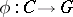there exists a unique homomorphism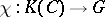that satisfies the condition.
This construction was first studied by A. Grothendieck for the categories of coherent and locally free sheaves on schemes in proving the Riemann–Roch theorem. See-functor in algebraic geometry. The groupis uniquely defined (up to isomorphism) and can be given by generators — to each object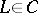there corresponds a generator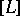— and by the relations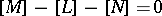for each exact sequenceIfis a topological space, then the Grothendieck group of the additive category of vector bundles overis an invariant of the space, studied in (topological)-theory. Ifis the category of non-degenerate symmetric bilinear forms on linear spaces over a field, thenis the Witt–Grothendieck group over(cf. Witt ring).